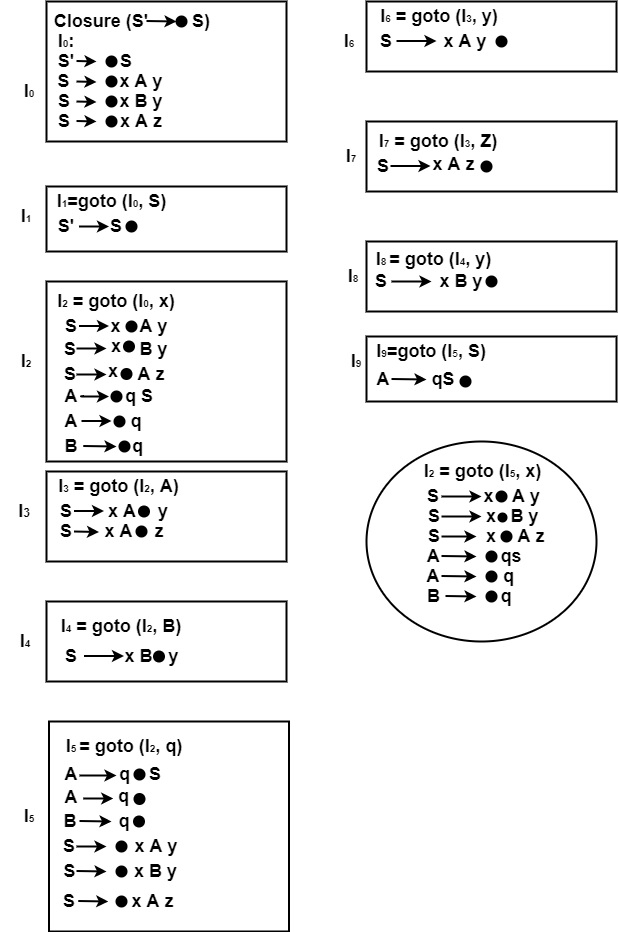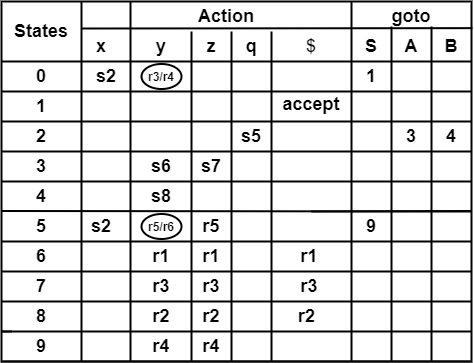# Construct SLR (1) parsing table for the following grammar S → x A y |x B y |x A z A → q s | q B → q

Compiler DesignProgramming LanguagesComputer Programming

#### Web Design for Beginners: Build Websites in HTML & CSS 2022

68 Lectures 8 hours

#### HTML5 & CSS3 Site Design

61 Lectures 6 hours

## Solution

Step1 − Construct Augmented Grammar

(0) S′ → S

(1) S → x A y

(2) S → x B y

(3) A → q S

(4) A → q

(5) B → q

Step2 − Find Closure & goto functions to construct LR (0) items. Here Boxes represent New States and Circles represent the repeating state.Step3 − Computation of FOLLOW

• S → x A y

FOLLOW(S) = {$} (1) Applying Rules (2a) of FOLLOW. Comparing S → x a y with A → α B β. ∴ FIRST(β) = FIRST(y) = {y} ∴ FOLLOW(A) = {y} (2) Rule (3) cannot be applied As, S → x A y cannot be compared with A → α B • S → x B y Applying Rules (2a) of FOLLOW. Comparing S → x B y with A → α B β. ∴ FIRST(β) = {y} ∴ FOLLOW(B) = {y} (3) Rule (3) cannot be applied. • S → x A y Applying Rules (2a) of FOLLOW. ∴ FIRST(β) = {z} ∴ FOLLOW(A) = {z} (4) Rule (3) cannot be applied. • A → q S Rule (2a) cannot be applied. As A → q s cannot be compared with A → α B β. Applying Rule (3) Comparing A → q s with A → α B ∴ A = A, α = q, B = S ∴ FOLLOW (S) = {FOLLOW(A)} (5) Rule (2a) and Rule (3) of FOLLOW cannot be applied on Production A → q and B → q. Combining Statement (1) to (5) FOLLOW(A) = {y, z} FOLLOW(S) = {$, y, z}

FOLLOW(B) = {y}

Step4 − Construction of SLR (1) Parsing Table